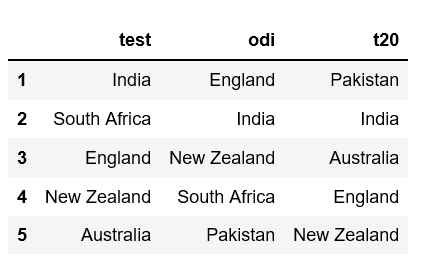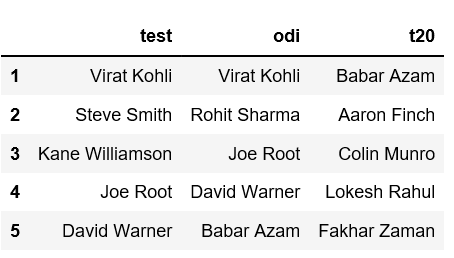# Python | Create a Pandas Dataframe from a dict of equal length lists

Given a dictionary of equal length lists, task is to create a Pandas DataFrame from it.

There are various ways of creating a DataFrame in Pandas. One way is to convert a dictionary containing lists of equal lengths as values. Let’s discuss how to create a Pandas Dataframe from a dict of equal length lists with help of examples.

Example #1: Given a dictionary which contains format of cricket as keys and list of top five teams as values.

 `# Import pandas package  ` `import` `pandas as pd ` ` `  `# Define a dictionary containing ICC rankings ` `rankings ``=` `{``'test'``: [``'India'``, ``'South Africa'``, ``'England'``, ` `                            ``'New Zealand'``, ``'Australia'``], ` `              ``'odi'``: [``'England'``, ``'India'``, ``'New Zealand'``, ` `                            ``'South Africa'``, ``'Pakistan'``], ` `               ``'t20'``: [``'Pakistan'``, ``'India'``, ``'Australia'``,  ` `                              ``'England'``, ``'New Zealand'``]} ` ` `  `# Convert the dictionary into DataFrame ` `rankings_pd ``=` `pd.DataFrame(rankings) ` ` `  `# Increment the index so that index  ` `# starts at 1 (starts at 0 by default)  ` `rankings_pd.index ``+``=` `1` ` `  `rankings_pd `

Output:Example #2: Given three lists `test_batsmen`, `odi_batsmen`, `t20_batsmen`. So we first need to convert this data into a dictionary and then convert the dictionary into DataFrame.

 `# Import pandas package  ` `import` `pandas as pd ` ` `  `# Lists of top 5 batsmen for each format ` `test_batsmen ``=` `[``'Virat Kohli'``, ``'Steve Smith'``, ``'Kane Williamson'``, ` `                                     ``'Joe Root'``, ``'David Warner'``] ` `odi_batsmen ``=` `[``'Virat Kohli'``, ``'Rohit Sharma'``, ``'Joe Root'``, ` `                            ``'David Warner'``, ``'Babar Azam'``] ` `t20_batsmen ``=` `[``'Babar Azam'``, ``'Aaron Finch'``, ``'Colin Munro'``, ` `                           ``'Lokesh Rahul'``, ``'Fakhar Zaman'``] ` ` `  `# Define a dictionary containing ICC rankings for batsmen ` `rankings_batsmen ``=` `{``'test'``: test_batsmen, ` `                      ``'odi'``: odi_batsmen, ` `                      ``'t20'``: t20_batsmen} ` ` `  `# Convert the dictionary into DataFrame ` `rankings_batsmen_pd ``=` `pd.DataFrame(rankings_batsmen) ` ` `  `# Increment the index so that index ` `# starts at 1 (starts at 0 by default)  ` `rankings_batsmen_pd.index ``+``=` `1` ` `  `rankings_batsmen_pd `

Output: